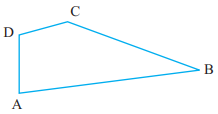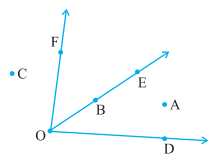### Chapter - 4 Basic Geometrical Ideas

Q
##### Basic Geometrical Ideas

Question:

1. Name the angles in the given figure.Solution: The name of angles are:

2. In the given diagram, name the point(s)

(a) In the interior of ∠DOE

(b) In the exterior of ∠EOF

(c) On ∠EOFSolution: (a) Point A is the point in the interior of ∠DOE.

(b) Point C, A and D are the point in the exterior of ∠EOF.

(c) Point B is the point on the ∠EOF.

3. Draw rough diagrams of two angles such that they have

(a) One point in common.

(b) Two points in common.

(c) Three points in common.

(d) Four points in common.

(e) One ray in common.

Solution: (a) O is the common point between ∠BOA and ∠DOC.(b) O and D are the common points between the ∠BOD and ∠COD.(c) O, E and D are the common points between the ∠BOD and ∠COD.(d) O, F, E and D are the common points between the ∠BOD and ∠COD.(e) OB is the common ray between the ∠AOB and ∠COB.